### Day 71 - Multiplying/Dividing Rational Expressions - 04.29.15

• Unit 6 Test
• next Friday, May 8th!

Questions

Bell Ringer
1. Find the excluded value(s) for the following: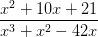1. 0

2. -7

3. 6

4. a, b, and c

5. none of the above

2. Simplify the following:1.2.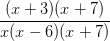3.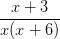5. none of the above

3. FInd the following product: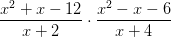1.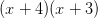2.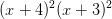3.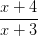4.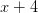5. none of the above

4. Find the following quotient: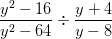1.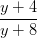2.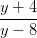3.4.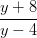5. none of the above

Review
• Fractions
• Operations with Fractions (+, -, *, /)
• Simplifying Fractions
• Rationals

Lesson

Exit Ticket
• Posted at the end of the block!

Essential Question(s)
• How can rational expressions be multiplied/divided?
Skills
1. Multiply/divide rational expressions.

#### In-Class Help Requests

Standard(s)
• CC.9-12.A.REI.2 Understand solving equations as a process of reasoning and explain the reasoning. Solve simple rational and radical equations in one variable, and give examples showing how extraneous solutions may arise.
• CC.9-12.N.RN.1 Extend the properties of exponents to rational exponents. Explain how the definition of the meaning of rational exponents follows from extending the properties of integer exponents to those values, allowing for a notation for radicals in terms of rational exponents. For example, we define 5^(1/3) to be the cube root of 5 because we want [5^(1/3)]^3 = 5^[(1/3) x 3] to hold, so [5^(1/3)]^3 must equal 5.
• CC.9-12.N.RN.2 Extend the properties of exponents to rational exponents. Rewrite expressions involving radicals and rational exponents using the properties of exponents.
• CC.8.G.8 Understand and apply the Pythagorean Theorem. Apply the Pythagorean Theorem to find the distance between two points in a coordinate system.

Mathematical Practice(s)
• #1 - Make sense of problems and persevere in solving them
• #2 - Reason abstractly and quantitatively
• #7 - Look for and make use of structure